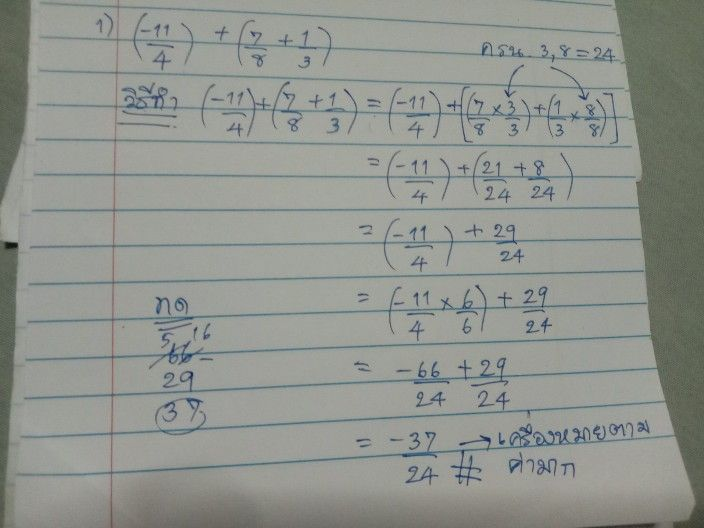เครื่องหมาย
โจทย์ปัญหา$2$ $939$ $12$ $\left(\dfrac {11} {4}\right)$ $-$ $+\left(\dfrac {7} {3}+\dfrac {1} {3}\right)$ $+\dfrac {1} {3}\right)$ $\left(-\dfrac {11} {4}\right)$ $+\left(\dfrac {9} {3}$ $\dfrac {11} {4}\times$
มัธยมต้น
คณิตศาสตร์
วิธีการแก้ปัญหาคุณครู Qanda - oilยังไม่เข้าใจใช่ไหม?
ถามคำถามกับครู Qanda